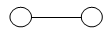### Kinetic Theory - CBSE Revision Notes

CBSE Class 11 PHYSICS

Revision Notes
CHAPTER 13
KINETIC THEORY

1. Molecular nature of matter and Behaviour of gases
2. Kinetic theory of an ideal gas
3. Law of equipartition of energy
4. Mean free path

Boyle’s Law: At constant temperature volume of given mass of gas is inversely proportional to its pressure. $V\alpha \frac{1}{P}$ or PV = cons tan t

Charle’s Law: At constant pressure volume of a given mass of gas is directly proportional to its absolute temperature.
*For 1° rise in temp.

Gay Lussac’sLaw: At constant volume, pressure of a given mass of gas is directly proportional to its absolute temp.
$\frac{p}{T}$ = cons tan t .
For ${1}^{\circ }C$ rise in temperature ${P}_{t}={P}_{0}\left(1+\frac{t}{273.15}\right)$

Ideal Gas Equation: for n mole of gas
PV=nRT,
for 1 mole , PV=RT
Universal gas constant: R =8.31 J $mo{l}^{-1}{K}^{-1}$
Boltzmann constant: ${k}_{B}=\frac{R}{{N}_{A}}$
Where ${k}_{B}$= Boltzmann constant, ${N}_{A}$= Avogadrosno.

Ideal gas: A gas which obeys gas law strictly is an ideal or perfect gas. The molecules of such a gas are of point size and there is no force of attraction between them.

Assumptions of Kinetic Theory of Gases
1.
All gases consist of molecules which are rigid, elastic spheres identical in all respect for a given gas.
2. The size of a molecule is negligible as compared with the average distance between two molecules.
3. During the random motion, the molecules collide with one another and with the wall of the vessel. The collisions are almost instantaneous.
4. The molecular density remains uniform throughout the gas.
5. The collisions are perfectly elastic in nature and there are no forces of attraction or repulsion between them.

Law of equipartion of energy: For any thermodynamical system in thermal equilibrium, the energy of the system is equally divided amongst its various degree of freedom and energy associated with each degree of freedom corresponding toeach molecule is $\frac{1}{2}{K}_{B}T$, where ${k}_{B}$ is the Boltzmann’s constant and T is absolute temperature.

• The law of equipartition of energy holds good for all degrees of freedom whether translational , rotational or vibrational.
• A monoatomic gas molecule has only translational kinetic energy
${E}_{t}+{E}_{r}$ $=\frac{1}{2}m{V}_{x}^{2}+\frac{1}{2}m{V}_{y}^{2}+\frac{1}{2}m{V}_{z}^{2}+\frac{3}{2}2KBT$
So a monoatomic gas molecule has only three (translational) degrees of freedom.
• In addition to translational kinetic energy, a diatomic molecule has two rotational

Kinetic energies

${E}_{t}+{E}_{r}$ $=\frac{1}{2}m{V}_{x}^{2}+\frac{1}{2}m{V}_{y}^{2}+\frac{1}{2}m{V}_{z}^{2}+\frac{1}{{21}_{y}}{W}_{y}^{2}+\frac{1}{{21}_{2}}{W}_{z}^{2}$Here the line joining the two atoms has been taken as x-axis about which there is no rotation. So, the degree of freedom of a diatomic molecule is 5, it does not vibrate.
At very high temperature, vibration is also activated due to which two extra degree of freedom emerge from vibrational energy. Hence at very high temperature degree of freedom of diatomic molecule is seven.
(Each translational and rotational degree of freedom corresponds to one mole of absorption of energy and has energy (1/2${k}_{B}$)